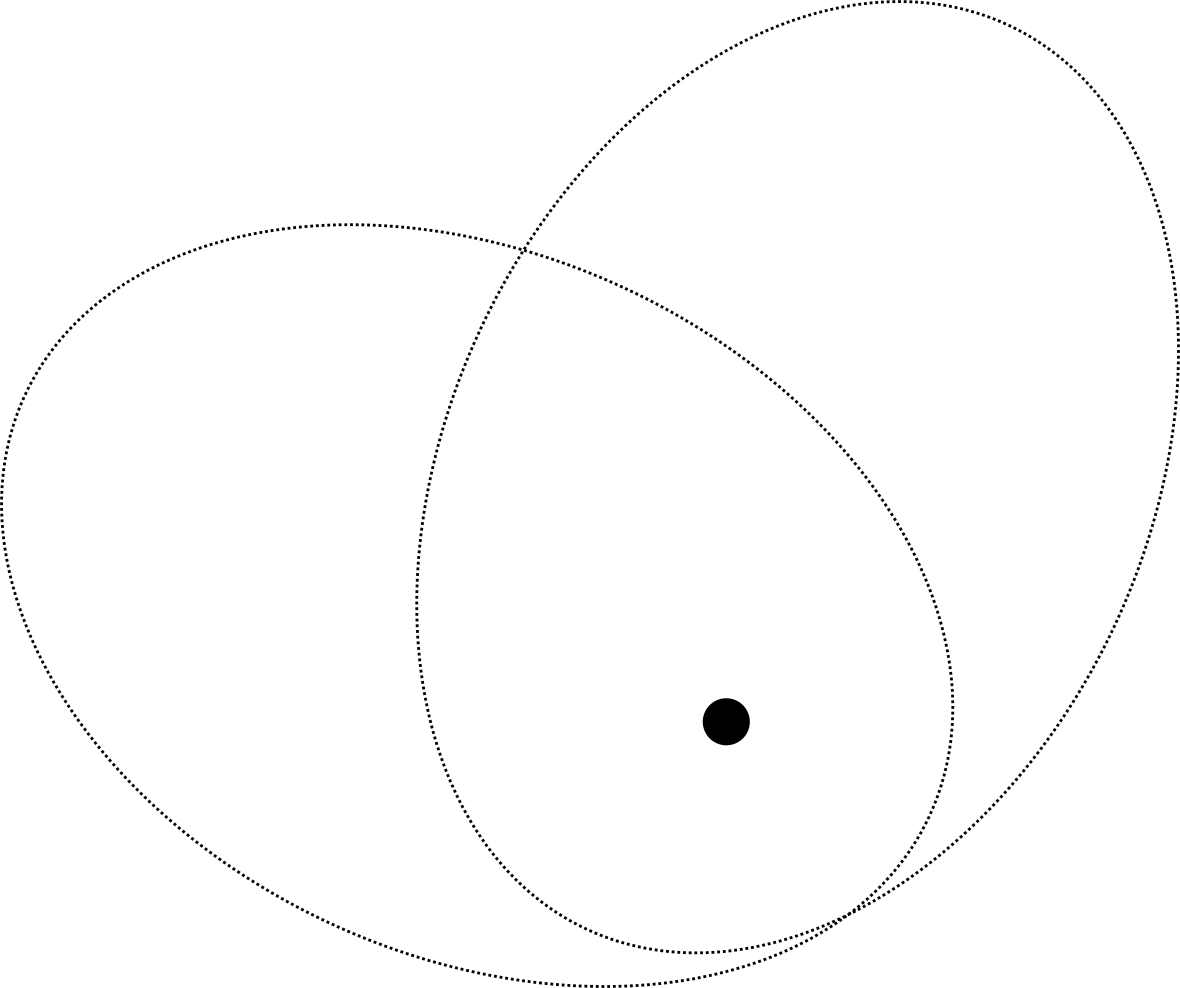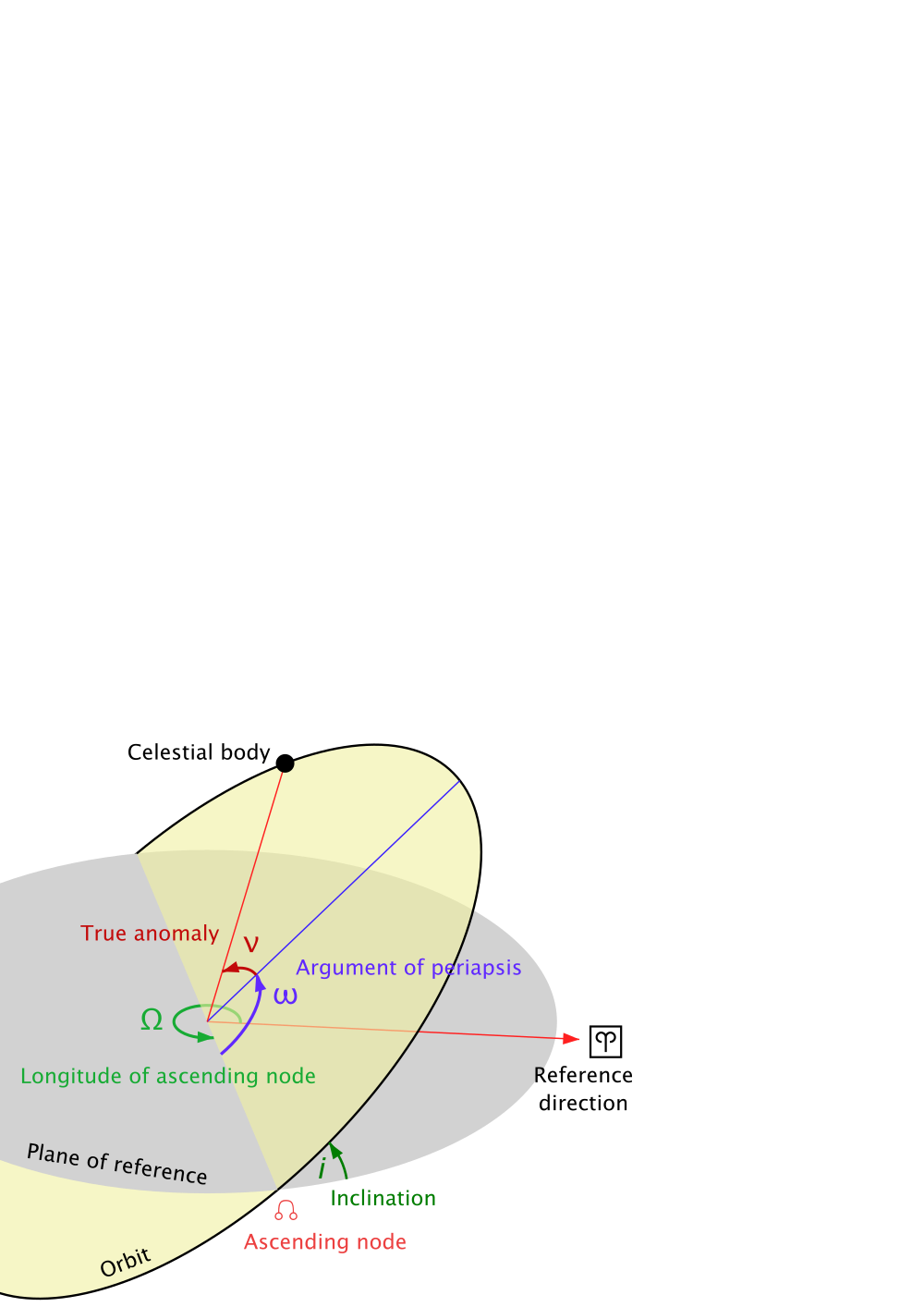# Stable solutions for general orbits in games

/ game physics

In the previous post, we saw how to do the math to get velocities and positions along an elliptical orbit. To translate this into game code though, there is still one problem we have to solve. So far we have spoken about apoapsis and periapsis as determining the shape of the ellipse that describes the orbit, but it doesn't uniquely identify the orbit itself. As you can see in the image below, the rendered orbits have the same periapsis and apoapsis, but they still do not coincide. The orbit has been rotated around the primary, as it were.To uniquely identify orbits, we need one more parameter, namely where the orbit reaches periapsis. We talk about the longitude of periapsis $\varpi$ with respect to some reference direction. For our code, we will assume the positive x-axis as our reference direction.

We assume that the satellite is at periapsis at some instant $t_{0}$, and we now know that the relative angle with the x-axis at periapsis is $\varpi$. This means we have our starting point, and from there we can use Kepler's equations to calculate the rest.

## Current position in elliptical orbit

In the previous post, we showed how to get the current true anomaly based on the elapsed time, the eccentricity, and mean anomaly of the orbit. As a reminder, the mean anomaly is the average change in the direction of the satellite around the primary, so it is just $2\pi$ (a full rotation) divided by the period. To find the period of an elliptical orbit, we look at our good old friend Kepler again. In particular, Kepler's Third Law says:

$$T = 2\pi \sqrt{\frac{a^3}{GM}}.$$

$T$ is the period in seconds, $a$ is the semi-major axis of our orbit as before, and $GM$ the gravitational constant multiplied with the mass of the primary. As with circular orbits before, as a game developer you have the option to choose these however you want to make things look as you want to. The mean anomaly is thus:

$$\mu = \frac{2\pi}{T} = \frac{2\pi}{2\pi \sqrt{\frac{a^3}{GM}}} = \frac{1}{\sqrt{\frac{a^3}{GM}}}$$

We now have a plan: whenever we create a new orbit in our game, with a primary, periapsis, apoapsis, $t_0$, and longitude of periapsis, we first need to calculate a few derivative properties: the eccentricity, the semi-major and semi-minor axes, the centre of the ellipse, and the mean anomaly. These values don't change. From that point onward, we use Kepler's equation to calculate the position ever frame.

The full code can be found below.

static class Constants {
public const double G = 1;
}

sealed class Body {
public Vector2 Position { get; }
public double Mass { get; }
}

sealed class Orbit {

public Orbit(
Body primary, double periapsis, double apoapsis, double tPer, double longitudePer) {
this.primary = primary;
this.tPer = tPer;

eccentricity = (apoapsis - periapsis) / (apoapsis + periapsis);
semiMajorAxis = 0.5 * (periapsis + apoapsis);
semiMinorAxis = semiMajorAxis * Math.Sqrt(1 - eccentricity * eccentricity);
ellipseCenterOffset = (periapsis - semiMajorAxis) * Vector2.UnitX;
meanAnomaly = 1 / Math.Sqrt(
semiMajorAxis * semiMajorAxis * semiMajorAxis / (Constants.G * primary.Mass));
longitudeTransform = Matrix2.CreateRotation(longitudePer);
}

public Vector2 PositionAtTime(double currentTime)
{
// Calculate the true anomaly using Kepler's equation.
var trueAnomaly = trueAnomalyAtTime(currentTime);

// We calculate the distance vector from the primary to the satellite
// under the assumption that longitude of periapsis is 0.
var preRotatedOffset = ellipseCenterOffset + new Vector2(
semiMajorAxis * Math.Cos(trueAnomaly),
semiMinorAxis * Math.Sin(trueAnomaly));

// We then rotate the point around based on the longitude of periapsis.
var rotatedOffset = longitudeTransform.Transform(preRotatedOffset);

// Finally, we translate this offset to be in the space of the primary.
return primary.Position + rotatedOffset;
}

private double trueAnomalyAtTime(double currentTime)
{
const numIterations = 30; // Change depending on expected eccentricities.

// Pre-calculate the current mean motion because it doesn't change.
var M = (currentTime - tPer) * meanAnomaly;
// Make an initial guess based on the eccentricity of the orbit.
var E = eccentricity > 0.8 ? Math.PI : M;

for (int i = 0; i < numIterations; i++)
{
var numerator = eccentricity * Math.Sin(E) + M - E;
var denominator = eccentricity * Math.Cos(E) - 1;
E = E - numerator / denominator;
}

return E;
}
}


That is a lot of code, but it is all derived directly from the equations we've seen over the past few posts. There is one new trick I have applied to deal with the longitude of periapsis though. Calculating offsets is hard when your ellipse is not axis-aligned. That is why for all the calculations, I just pretend our primary is the origin, and the periapsis lies along the positive x-axis. When we calculated the difference vector from the primary to the satellite in this normalized space, we transform it by first rotating around the origin, and then calculating the final position by adding the rotated difference vector to the primary's position.

## The third dimension

We spent five posts getting to the point of being able to describe an elliptical orbit in code. Surely, doing this in three dimensions will be much harder! Nothing less would be the case. All the equations still hold up in three dimensions. As a matter of fact, we have assumed that the physical rules of a 3D world hold up, and that we are merely working in a two-dimensional projection of the 3D world. This is also exactly why the step from 2D to 3D is relatively straightforward: we just have to put our code to work in a specific plane in that 3D world.

In orbital mechanics, we don't only have a reference for the longitude of periapsis, we also have a reference plane. In games we could easily pick the xy-plane, in the real world it is often the plane that is set out by the primary's equator. Our orbit will either coincide with the plane, or it will pass the plane through two points. The angle the orbit makes with the reference plane is called the inclination. The points in which the satellite passes through the reference plane are called the ascending node (when the orbit goes from below the plane to above it) and descending node (when the orbit goes in the other direction).

Earlier we used the longitude of periapsis to determine the orientation of the orbit, but in practice, we don't actually use that parameter. Instead, we use something called the longitude of the ascending node $\Omega$. Instead of measuring the angle between the reference direction and the periapsis, we measure the angle between the reference direction and the ascending node. To then fix where the periapsis is, we express the position of the periapsis as the argument of periapsis $\omega$ (yes they're both omegas, I didn't choose the symbols). The argument of periapsis is the angle between the ascending node and the periapsis measured in the orbital plane. The reason it is chosen this way is that with third dimensions, the periapsis no longer necessarily lies in the reference plane, meaning that calculating the angle between a reference direction and the periapsis becomes an undefined problem.To implement the longitude of periapsis in our code, we used a transformation that kept the math simple by moving the primary to the origin, and the periapsis on the positive x-axis. We can do the same thing for inclination. Remember that transformations are always applied "backwards" or right to left, so the steps in the code become:

• Rotate rotatedOffset around the z-axis (so it rotates in the reference plane xy) by the argument of periapsis. Our orbit now represents how the orbit looks with the orbital plane as reference plane, and the ascending node at the positive x-axis.
• Rotate rotatedOffset around the x-axis by the inclination. We now have our actual orbit, but the ascending node is not yet in the right position.
• Rotate rotatedOffset around the z-axis, since the xy-plane now the actual reference plane, by the longitude of the ascending node.

Since these three rotations never change, we can precalculate the resulting matrix, and just translate our result once. That makes our final code:

static class Constants {
public const double G = 1;
}

sealed class Body {
public Vector2 Position { get; }
public double Mass { get; }
}

sealed class Orbit {

public Orbit(
Body primary, double periapsis, double apoapsis,
double tPer, double inclination, double longitudeAscNode, double argPeriapsis) {
this.primary = primary;
this.tPer = tPer;

eccentricity = (apoapsis - periapsis) / (apoapsis + periapsis);
semiMajorAxis = 0.5 * (periapsis + apoapsis);
semiMinorAxis = semiMajorAxis * Math.Sqrt(1 - eccentricity * eccentricity);
ellipseCenterOffset = (periapsis - semiMajorAxis) * Vector2.UnitX;
meanAnomaly = 1 / Math.Sqrt(
semiMajorAxis * semiMajorAxis * semiMajorAxis / (Constants.G * primary.Mass));
orbitTransform = createOrbitalTransformMatrix(
inclination, longitudeAscNode, argPeriapsis);
}

private Matrix3 createOrbitalTransformMatrix(
double inclination, double longitudeAscNode, double argPeriapsis) {
// Rotate the periapsis in position assuming the ascending node is the
// positive x-axis.
var periapsisTransform = Matrix3.CreateAxisRotation(Vector3.UnitZ, argPeriapsis);
// Rotate the orbit around the x-axis to give it its inclination.
// We use the negative rotation here to make sure the x-axis is the
// ascending node.
var inclinationTransform = Matrix3.CreateAxisRotation(Vector3.UnitX, -inclination)
// Finally, rotate the orbit so that the ascending node is in the right
// position as well.
var ascNodeTransform = Matrix3.CreateAxisRotation(Vector3.UnitZ, longitudeAscNode);

// Transformations are applied right to left, so make sure we multiply
// them in that order.
return ascNodeTransform * inclinationTransform * periapsisTransform;
}

public Vector2 PositionAtTime(double currentTime)
{
// Calculate the true anomaly using Kepler's equation.
var trueAnomaly = trueAnomalyAtTime(currentTime);

// We calculate the distance vector from the primary to the satellite
// under the assumption that longitude of periapsis is 0.
// Note that the z-coordinate is 0, since the orbit is in the xy-plane.
var preRotatedOffset = ellipseCenterOffset + new Vector3(
semiMajorAxis * Math.Cos(trueAnomaly),
semiMinorAxis * Math.Sin(trueAnomaly),
0);

// We then rotate the point in 3D space to apply inclination and the
// correct orientation of the periapsis and ascending node.
var rotatedOffset = orbitTransform.Transform(preRotatedOffset);

// Finally, we translate this offset to be in the space of the primary.
return primary.Position + rotatedOffset;
}

private double trueAnomalyAtTime(double currentTime)
{
const numIterations = 30; // Change depending on expected eccentricities.

// Pre-calculate the current mean motion because it doesn't change.
var M = (currentTime - tPer) * meanAnomaly;
// Make an initial guess based on the eccentricity of the orbit.
var E = eccentricity > 0.8 ? Math.PI : M;

for (int i = 0; i < numIterations; i++)
{
var numerator = eccentricity * Math.Sin(E) + M - E;
var denominator = eccentricity * Math.Cos(E) - 1;
E = E - numerator / denominator;
}

return E;
}
}


For bonus points, since all the transformations are rotations, you could switch to using a quaternion instead to speed up that step, but that part is left as an exercise to the reader.